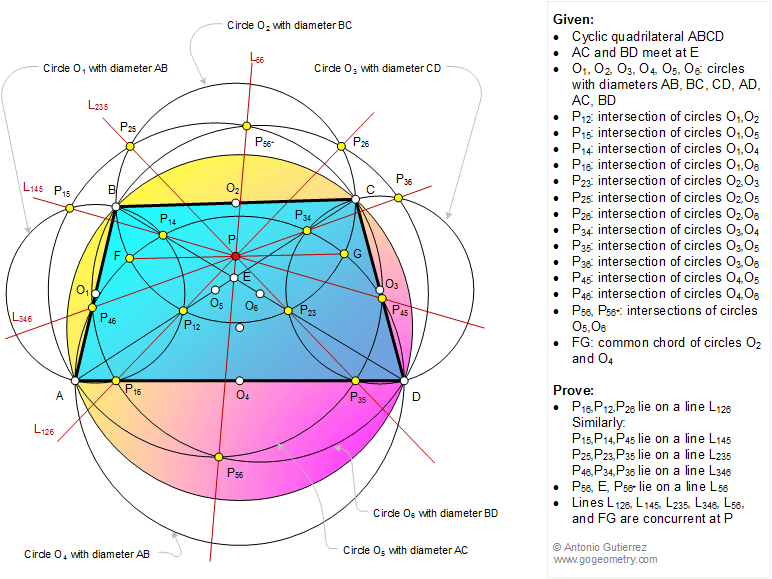# Geometry Problem 1176: Cyclic Quadrilateral, Diagonals, Six Diameters, Circles, Collinear Points, Concurrent Lines. Level: High School, College, Mathematics Education

< PREVIOUS PROBLEM  |  NEXT PROBLEM >

 The figure shows a cyclic quadrilateral ABCD, AC and BD meet at E. O1, O2, O3, O4, O5, O6 are circles with diameters AB, BC, CD, AD, AC, and BD, respectively. P12, P14, P15, P16, P23, P25, P26, P34, P35, P36, P45, P46, P56, P56* are the points of intersection of circles O1 and O2, O1 and O4, O1 and O5, O1 and O6, O2 and O3, O2 and O5, O2 and O6, O3 and O4, O3 and O5, O3 and O6, O4 and O5, O4 and O6, and O5 and O6, respectively. FG is the common chord of circles O2 and O4. Prove that (1) P16, P12, and P26 are collinear (lie on a line L126), similarly P15, P14, and P45 are collinear (lie on a line L145), P25, P23, and P35 are collinear (lie on a line L235), P46, P34, and P36 are collinear (lie on a line L346); (2) P56, E, and P56* are collinear (line on a line L56); (3) Lines L126, L145, L235, L346, L56, and FG are concurrent at P.Home | Search | Geometry | Problems | All Problems | Open Problems | Visual Index | 10 Problems | Problems Art Gallery |  Art | 1171-1180 | Cyclic Quadrilateral | Quadrilateral | Circle | Chord | Common Chord | Intersecting Circles | Collinear Points | Concurrent linesLast updated: Jan 11, 2016 by Antonio Gutierrez